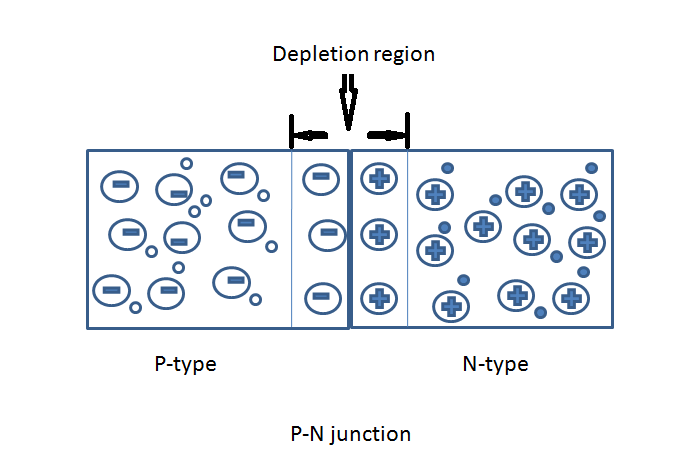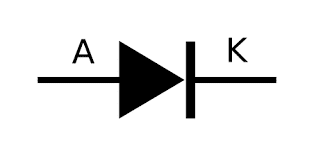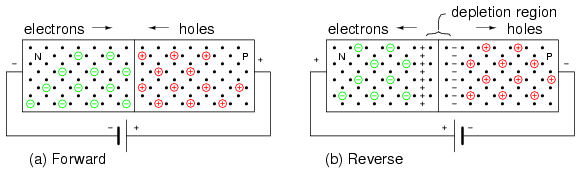Pn junction diode is one of the most basic diodes. It is used in the construction of transistors, FET, Gates and many other types of integrated circuits. These are the easiest and basic diodes available in and use PN junction characteristics like depletion layer, diffusion and drift current. We prefer to read our article on PN Junction first before reading this guide for maximum understanding.

But let us also give you an idea of diodes. A Diode is an electronic device that conducts current in one direction i.e. either in the forward direction or in the reverse direction at a moment of given time. We hope you all have read and understood diodes working but if you want to brush up the topic again, we have written a very simple and easy understanding Diodes basic guide for you.

[Image source]

## What is a PN junction diodeA PN Junction diode is a 2 terminal device fabricated by joining P-type and N-type semiconductors chemically (image given above) and . It allows electric current to pass only in one direction while blocks in the other direction. The direction of the current depends upon the biasing of the diode.

If the diode is Forward biased it will allow the current to pass through the circuit but if the diode is reverse biased, it will block the current.

[su_note note_color=”#ff0000″ text_color=”#ffffff”]Extra Marks: PN Junction Diode is also referred to as PN Junction. A PN junction with two metallic contacts is known as PN junction diode or semiconductor diode.[/su_note]

The Schematic representation or basic symbol of PN Junction diode is given below:## How PN Junction Diode Works?

To understand the working of a p-n junction diode, we connect it to an external voltage over a range of 0v, 5v, 10v, and so on.  This will helps us in determining the variation in the current passing through the p-n junction with increasing voltage levels.

We usually connect two metallic contacts at the ends of the p-n junction to apply external voltage.

As we know diode is a specialized electronic component with two electrodes called the anode and cathode conducts current in one direction. As shown in the figure above, the direction of the arrow indicates the direction of the conventional current.

### Biasing of PN Junction:

The process of applying an external voltage to the p-n junction diode is known as the biasing. We generally bias p-n junction in the following ways. The full explanation of Biasing a diode is given below with VI characteristics of PN Junction Diode.

• Forward bias: In forward bias negative terminal is connected to n-type material and the positive terminal is connected to p-type material across the diode shows the decrease in the built-in potential barrier.
• Reverse bias: In reverse bias negative terminal is connected to p-type material and the positive terminal is connected to n-type material across the diode shows the increase in the built-in potential barrier.
• Zero bias: In zero bias no external voltage is applied.

## PN Junction diode Biasing Explanation

In the modern electronics p-n junction possesses special properties that are useful in many applications. We can bias the PN junction in the following ways by applying an external voltage. 3 possible biasing conditions and 2 operating regions for the typical p-n junction diode are explained below.[Image source]

### (i) Zero bias diode:

In zero bias or thermal equilibrium state, junction potential provides higher potential energy to the holes on the p-side than the n-side. When we short the terminals of the junction diode, few majority carriers in the p-side with maximum threshold energy starts travel across the depletion region. With the help of majority charge carriers, the current start flowing in the diode known as forward current.

Similarly, minority charge carriers in the n-side move across the depletion region in the reverse direction. This movement of minority charge carriers give rise to a reverse current. Due to this, the potential barrier opposes the movement of electrons and holes across the junction. But it permits the minority carriers to drift across the p-n junction.

Therefore, the potential barrier helps minority charge carriers in p-type and n-type to drift across the p-n junction. After this when the majority charge carriers are equal and both are moving in a reverse direction, the equilibrium is established. This indicates the zero current flowing in the circuit. That’s why the junction is said to be in a state of dynamic equilibrium.

### (ii) Forward biased diode:

Forward biasing of a p-n junction diode is very simple. To forward bias a PN junction diode, we connect its p-side to the positive terminal and n-side to the negative terminal of the battery or other current source.

When an external voltage is applied, the majority charge carriers in N-side and P-side regions attract towards the PN junction. This results in the reduction of the width of the depletion layer due to the diffusion of the majority charge carriers. So an electric field gets induced in the direction opposite to that of the incorporated field.

When the forward bias is greater than the built-in potential, the depletion region becomes much thinner. Because of this a large number of majority charge carriers cross the PN junction and conducts an electric current. The current flowing up to built-in potential (potential across the depletion region in thermal equilibrium) is known as KNEE current.

### (iii) Reverse bias diode:

Forward biasing is completely opposite to the forward biasing. In reverse bias condition, we connect voltage sources’ positive terminal to the n-side and negative terminal to the p-side of the p-n junction diode.

When an external voltage is applied, the positive terminal attracts the electrons. This makes electrons move away from the junction in the N-side. Similarly, the negative terminal attracts the holes, and holes move away from the junction in the P side.

This results in the increment of the width of the depletion region and so the potential barrier. With the increase in the potential barrier width, the electric field at the junction also starts increasing, and the p-n junction act as a resistor.

Minority charge carriers generated at the depletion region cause the small current also known as the Leakage Current in the junction diode. This indicates that the increase in the width of the depletion layer presents a high impedance path which acts as an insulator.

When the reverse bias potential across the p-n junction diode increases, the reverse breakdown voltage occurs. This reverse breakdown voltage causes the diode current to be controlled by an external circuit. If we increase reverse bias further, the PN junction diode becomes short circuit due to overheating of the circuit and maximum circuit current flows in the PN junction diode.

## PN Junction Diode VI Characteristics

PN junction diode shows zero resistance in the forward direction and infinite resistance in the reverse direction. i.e., it is not a perfect diode. Before using this diode, it is necessary to know a little about its characteristics and properties with forward bias and reverse bias.

To know about its characteristics we plot a graph between voltage and current along the x-axis and y-axis which shows the behavior of PN junction diode in forward biasing and in reverse biasing. X-axis represents Voltage and Y-axis represents Currents in milliampere (mA).

### (i) PN Junction diode forward Biased characteristics:

As shown in the figure below, the VI characteristics of junction diode are not linear (straight line) but it rises linearly to a point and then rises exponentially. This nonlinear characteristic indicates that the resistance is not constant during the operation of the PN junction.

When forward biasing is applied to the diode; initially; a small amount of current starts flowing until the point of Knee Voltage. This initial current is also known as infinite current and is considered negligible. It happens due to the low impedance of the junction diode. This current starts flowing above the knee point with a small amount of external potential.

If we increase the current further, it may damage the diode. To overcome this we use a load resistor that controls the flow of current and save the device from damaging.

### (ii) PN Junction diode reverse Biased characteristics:

In this type of biasing the current is low till breakdown is reached and hence the diode is like an open circuit. The characteristic curve of this diode is shown in the fourth quadrant of the given figure above. When the input voltage of the reverse bias has reached the breakdown voltage, reverse current increases enormously. In reverse direction, a perfect diode would not allow any current to flow.

## Pn junction diode Equation:

The PN junction diode equation for an ideal diode is given below:

I = IS[exp(eV/KBT) – 1]

Here,

IS = reverse saturation current

e = charge of electron

KB = Boltzmann constant

T = temperature

For a normal PN junction diode, the equation becomes

I = IS[exp(eV/ɳKBT) – 1]

Here, ɳ = emission co-efficient, which is a number between 1 and 2, which typically increases as the current  increases.